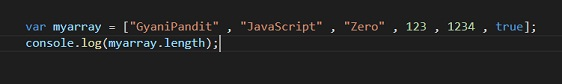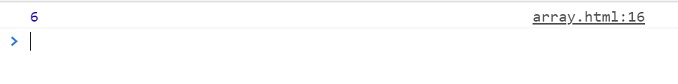# javascript array length

Javascript Array Length

By length of the array, we just mean the number of elements in the array. So, for getting the length of the array, you can use the length property. Remember that the length of the array simply means the number of elements in the array.

Let’s have a look at the below program, where we are trying to get the length of the array.Lets now have a look at the output for the above program –As you can see that there are 6 elements in the array, so the length of the array is 6. Notice how are we using the length property. We are writing the variable name, followed by the dot, and then we are writing length, and this is how we are getting the length of the array. We can make use of the length property, whenever we need the length of the array.